# Primal-Dual Proximal Splitting¶

$\newcommand{\dotp}{\langle #1, #2 \rangle}$ $\newcommand{\enscond}{\lbrace #1, #2 \rbrace}$ $\newcommand{\pd}{ \frac{ \partial #1}{\partial #2} }$ $\newcommand{\umin}{\underset{#1}{\min}\;}$ $\newcommand{\umax}{\underset{#1}{\max}\;}$ $\newcommand{\umin}{\underset{#1}{\min}\;}$ $\newcommand{\uargmin}{\underset{#1}{argmin}\;}$ $\newcommand{\norm}{\|#1\|}$ $\newcommand{\abs}{\left|#1\right|}$ $\newcommand{\choice}{ \left\{ \begin{array}{l} #1 \end{array} \right. }$ $\newcommand{\pa}{\left(#1\right)}$ $\newcommand{\diag}{{diag}\left( #1 \right)}$ $\newcommand{\qandq}{\quad\text{and}\quad}$ $\newcommand{\qwhereq}{\quad\text{where}\quad}$ $\newcommand{\qifq}{ \quad \text{if} \quad }$ $\newcommand{\qarrq}{ \quad \Longrightarrow \quad }$ $\newcommand{\ZZ}{\mathbb{Z}}$ $\newcommand{\CC}{\mathbb{C}}$ $\newcommand{\RR}{\mathbb{R}}$ $\newcommand{\EE}{\mathbb{E}}$ $\newcommand{\Zz}{\mathcal{Z}}$ $\newcommand{\Ww}{\mathcal{W}}$ $\newcommand{\Vv}{\mathcal{V}}$ $\newcommand{\Nn}{\mathcal{N}}$ $\newcommand{\NN}{\mathcal{N}}$ $\newcommand{\Hh}{\mathcal{H}}$ $\newcommand{\Bb}{\mathcal{B}}$ $\newcommand{\Ee}{\mathcal{E}}$ $\newcommand{\Cc}{\mathcal{C}}$ $\newcommand{\Gg}{\mathcal{G}}$ $\newcommand{\Ss}{\mathcal{S}}$ $\newcommand{\Pp}{\mathcal{P}}$ $\newcommand{\Ff}{\mathcal{F}}$ $\newcommand{\Xx}{\mathcal{X}}$ $\newcommand{\Mm}{\mathcal{M}}$ $\newcommand{\Ii}{\mathcal{I}}$ $\newcommand{\Dd}{\mathcal{D}}$ $\newcommand{\Ll}{\mathcal{L}}$ $\newcommand{\Tt}{\mathcal{T}}$ $\newcommand{\si}{\sigma}$ $\newcommand{\al}{\alpha}$ $\newcommand{\la}{\lambda}$ $\newcommand{\ga}{\gamma}$ $\newcommand{\Ga}{\Gamma}$ $\newcommand{\La}{\Lambda}$ $\newcommand{\si}{\sigma}$ $\newcommand{\Si}{\Sigma}$ $\newcommand{\be}{\beta}$ $\newcommand{\de}{\delta}$ $\newcommand{\De}{\Delta}$ $\newcommand{\phi}{\varphi}$ $\newcommand{\th}{\theta}$ $\newcommand{\om}{\omega}$ $\newcommand{\Om}{\Omega}$

This tour explores a primal-dual proximal splitting algorithm, with application to imaging problems.

In :
using NtToolBox
using PyPlot

WARNING: Method definition ndgrid(AbstractArray{T<:Any, 1}) in module NtToolBox at /Users/quentin/.julia/v0.5/NtToolBox/src/ndgrid.jl:3 overwritten at /Users/quentin/.julia/v0.5/NtToolBox/src/ndgrid.jl:3.
WARNING: Method definition ndgrid(AbstractArray{#T<:Any, 1}, AbstractArray{#T<:Any, 1}) in module NtToolBox at /Users/quentin/.julia/v0.5/NtToolBox/src/ndgrid.jl:6 overwritten at /Users/quentin/.julia/v0.5/NtToolBox/src/ndgrid.jl:6.
WARNING: Method definition ndgrid_fill(Any, Any, Any, Any) in module NtToolBox at /Users/quentin/.julia/v0.5/NtToolBox/src/ndgrid.jl:13 overwritten at /Users/quentin/.julia/v0.5/NtToolBox/src/ndgrid.jl:13.
WARNING: Method definition ndgrid(AbstractArray{#T<:Any, 1}...) in module NtToolBox at /Users/quentin/.julia/v0.5/NtToolBox/src/ndgrid.jl:19 overwritten at /Users/quentin/.julia/v0.5/NtToolBox/src/ndgrid.jl:19.
WARNING: Method definition meshgrid(AbstractArray{T<:Any, 1}) in module NtToolBox at /Users/quentin/.julia/v0.5/NtToolBox/src/ndgrid.jl:33 overwritten at /Users/quentin/.julia/v0.5/NtToolBox/src/ndgrid.jl:33.
WARNING: Method definition meshgrid(AbstractArray{#T<:Any, 1}, AbstractArray{#T<:Any, 1}) in module NtToolBox at /Users/quentin/.julia/v0.5/NtToolBox/src/ndgrid.jl:36 overwritten at /Users/quentin/.julia/v0.5/NtToolBox/src/ndgrid.jl:36.
WARNING: Method definition meshgrid(AbstractArray{#T<:Any, 1}, AbstractArray{#T<:Any, 1}, AbstractArray{#T<:Any, 1}) in module NtToolBox at /Users/quentin/.julia/v0.5/NtToolBox/src/ndgrid.jl:44 overwritten at /Users/quentin/.julia/v0.5/NtToolBox/src/ndgrid.jl:44.


## Convex Optimization with a Primal-Dual Scheme¶

In this tour we use the primal-dual algorithm detailed in:

Antonin Chambolle and Thomas Pock A First-order primal-dual algorithm for convex problems with application to imaging, Journal of Mathematical Imaging and Vision, Volume 40, Number 1 (2011), 120-145

One should note that there exist many other primal-dual schemes.

We consider general optimization problems of the form $$\umin{f} F(K(f)) + G(f)$$ where $F$ and $G$ are convex functions and $K : f \mapsto K(f)$ is a linear operator.

For the primal-dual algorithm to be applicable, one should be able to compute the proximal mapping of $F$ and $G$, defined as: $$\text{Prox}_{\gamma F}(x) = \uargmin{y} \frac{1}{2}\norm{x-y}^2 + \ga F(y)$$ (the same definition applies also for $G$).

The algorithm reads: $$g_{k+1} = \text{Prox}_{\sigma F^*}( g_k + \sigma K(\tilde f_k)$$ $$f_{k+1} = \text{Prox}_{\tau G}( f_k-\tau K^*(g_k) )$$ $$\tilde f_{k+1} = f_{k+1} + \theta (f_{k+1} - f_k)$$

The dual functional is defined as $$F^*(y) = \umax{x} \dotp{x}{y}-F(x).$$ Note that being able to compute the proximal mapping of $F$ is equivalent to being able to compute the proximal mapping of $F^*$, thanks to Moreau's identity: $$x = \text{Prox}_{\tau F^*}(x) + \tau \text{Prox}_{F/\tau}(x/\tau)$$

It can be shown that in the case $\theta=1$, if $\sigma \tau \norm{K}^2<1$, then $f_k$ converges to a minimizer of the original minimization of $F(K(f)) + G(f)$.

More general primal-dual schemes have been developped, see for instance

L. Condat, A primal-dual splitting method for convex optimization involving Lipschitzian, proximable and linear composite terms, J. Optimization Theory and Applications, 2013, in press.

## Inpainting Problem¶

We consider a linear imaging operator $\Phi : f \mapsto \Phi(f)$ that maps high resolution images to low dimensional observations. Here we consider a pixel masking operator, that is diagonal over the spacial domain.

In :
?crop

search: macroexpand cross macro recvfrom cumprod cumprod! @fetchfrom

Couldn't find crop
Perhaps you meant chop, drop, cross, cor, cool, chol, chomp, cmp, copy or cos

Out:

No documentation found.

Binding crop does not exist.

In :
512^2

Out:
262144
In :
n=256
s1,s2=size(f0)
f0 = f0[Base.div(s1-n,2):Base.div(s1-n,2)+n-1, Base.div(s2-n,2):Base.div(s2-n,2)+n-1];


Display it.

In :
imageplot(f0);We consider here the inpainting problem. This simply corresponds to a masking operator.

Load a random mask $\La$.

In :
rho = .8
Lambda = rand(n,n).>rho;


Masking operator $\Phi$.

In :
Phi = f -> f.*Lambda;


Compute the observations $y=\Phi f_0$.

In :
y = Phi(f0);


Display it.

In :
imageplot(y);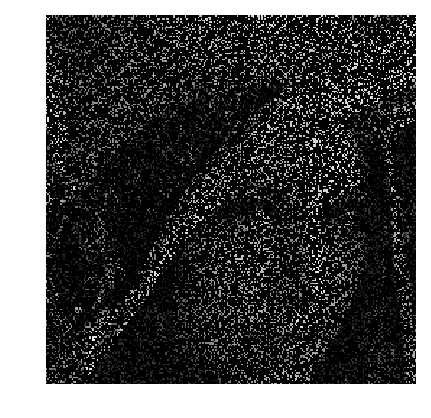## Total Variation Regularization under Constraints¶

We want to solve the noiseless inverse problem $y=\Phi f$ using a total variation regularization: $$\umin{ y=\Phi f } \norm{\nabla f}_1$$

This can be recasted as the minimization of $F(K(f)) + G(f)$ by introducing $$G(f)=i_H(f), \quad F(u)=\norm{u}_1 \qandq K=\nabla,$$ where $H = \enscond{x}{\Phi(x)=y}$ is an affine space, and $i_H$ is the indicator function $$i_H(x) = \choice{ 0 \qifq x \in H, \\ +\infty \qifq x \notin H. }$$

Shorcut for the operators.

In :
K  = f -> Grad(f)
KS = u -> -Div(u);


Shortcut for the TV norm.

In :
Amplitude = u -> sqrt(sum(u.^2,3));
F = u -> sum(sum(Amplitude(u)));


The proximal operator of the vectorial $\ell^1$ norm reads $$\text{Prox}_{\lambda F}(u) = \max\pa{0,1-\frac{\la}{\norm{u_k}}} u_k$$

In :
ProxF = (u,lambda) -> max(0,1-lambda./repeat(Amplitude(u), outer=(1, 1, 2))).*u;


Display the thresholding on the vertical component of the vector.

In :
figure(figsize=(10,10))
ax = gca(projection="3d")
t = -linspace(-2,2, 201);
(Y,X) = meshgrid(t,t);
U = cat(3,Y,X);
V = ProxF(U,1);
# 3D display
surf(V[:,:,1]);
set_cmap("jet")
#ax[:view](-150, 40)
#gca()[:invert_zaxis]()
ax[:view_init](-150, 40)

#axis("tight");
#camlight;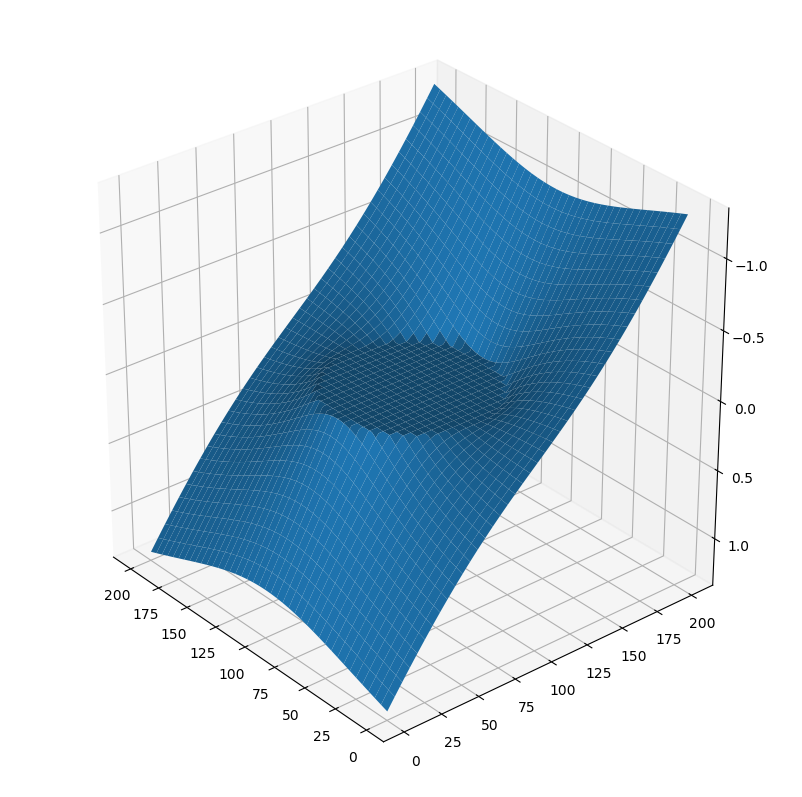For any 1-homogeneous convex functional, the dual function is the indicator of a convex set. For the $\ell^1$ norm, it is the indicator of the $\ell^\infty$ ball $$F^* = i_{\norm{\cdot}_\infty \leq 1} \qwhereq \norm{u}_\infty = \umax{i} \norm{u_i}.$$

The proximal operator of the dual function is hence a projector (and it does not depend on $\sigma$ ) $$\text{Prox}_{\sigma F^*}(u) = \text{Proj}_{\norm{\cdot}_\infty \leq 1}(u).$$

A simple way to compute the proximal operator of the dual function $F^*$, we make use of Moreau's identity: $$x = \text{Prox}_{\tau F^*}(x) + \tau \text{Prox}_{F/\tau}(x/\tau)$$

In :
ProxFS = (y,sigma) -> y-sigma*ProxF(y/sigma,1/sigma);


Display this dual proximal on the vertical component of the vector.

In :
V = ProxFS(U,1);
# display
surf(V[:,:,1]);
set_cmap("jet")
gca()[:view_init](-150, 40)
axis("tight");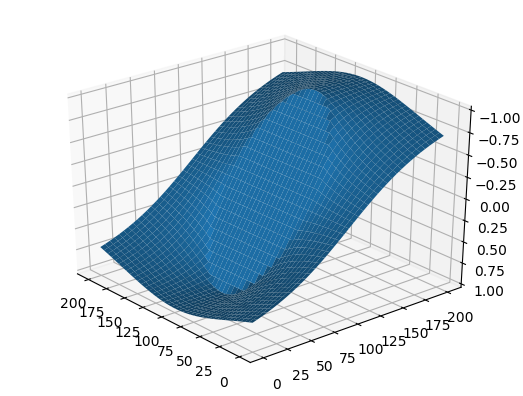The proximal operator of $G = i_H$ is the projector on $H$. In our case, since $\Phi$ is a diagonal so that the projection is simple to compute $$\text{Prox}_{\tau G}(f) = \text{Proj}_{H}(f) = f + \Phi(y - \Phi(f))$$

In :
ProxG = (f,tau) -> f + Phi(y - Phi(f));


## Primal-dual Total Variation Regularization Algorithm¶

Now we can apply the primal dual scheme to the TV regularization problem.

We set parameters for the algorithm. Note that in our case, $L=\norm{K}^2=8$. One should has $L \sigma \tau < 1$.

In :
L = 8;
sigma = 10;
tau = .9/(L*sigma);
theta = 1;


Initialization, here |f| stands for the current iterate $f_k$, |g| for $g_k$ and |f1| for $\tilde f_k$.

In :


Out:
(::#3) (generic function with 1 method)
In :
f = y;
g = K(y)*0;
f1 = f;

ArgumentError: unable to check bounds for indices of type Tuple{Array{Int64,1},Array{Int64,1}}

in checkindex at ./abstractarray.jl:378 [inlined]
in checkbounds_indices at ./abstractarray.jl:309 [inlined]
in checkbounds at ./abstractarray.jl:270 [inlined]
in checkbounds at ./abstractarray.jl:284 [inlined]
in _getindex at ./multidimensional.jl:270 [inlined]
in getindex at ./abstractarray.jl:752 [inlined]
in (::##3#4)(::Array{Float32,2}) at ./In:1

Example of one iterations.

In :
fold = f;
g = ProxFS( g+sigma*K(f1), sigma);
f = ProxG(  f-tau*KS(g), tau);
f1 = f + theta * (f-fold);

UndefVarError: g not defined


Exercise 1

Implement the primal-dual algorithm. Monitor the evolution of the TV energy $F(K(f_k))$ during the iterations. Note that one always has $f_k \in H$ so that the iterates satisfies the constraints.

In :
exo1()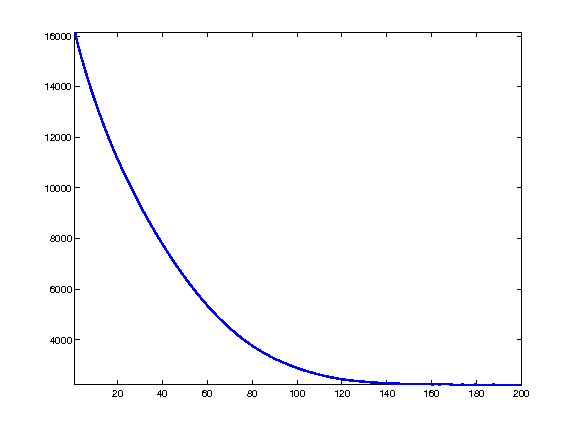In :
%% Insert your code here.


Display inpainted image.

In :
clf;
imageplot(f);Exercise 2

Use the primal dual scheme to perform regularization in the presence of noise $$\umin{\norm{y-\Phi(f)} \leq \epsilon} \norm{\nabla f}_1.$$

In :
exo2()

In :
%% Insert your code here.


## Inpainting Large Missing Regions¶

It is possible to consider a more challening problem of inpainting large missing regions.

To emphasis the effect of the TV functional, we use a simple geometric image.

In :
n = 64;
name = 'square';


We remove the central part of the image.

In :
a = 4;
Lambda = ones(n);
Lambda(end/2-a:end/2+a,:) = 0;
Phi = @(f)f.*Lambda;


Display.

In :
clf;
imageplot(f0, 'Original', 1,2,1);
imageplot(Phi(f0), 'Damaged', 1,2,2);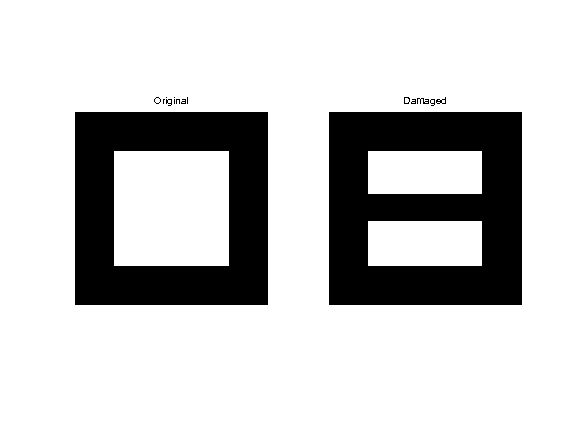Exercise 3

Display the evolution of the inpainting process.

In :
exo3()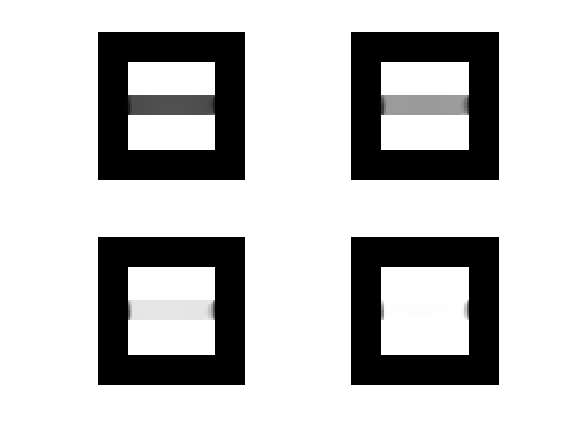In :
%% Insert your code here.

In :